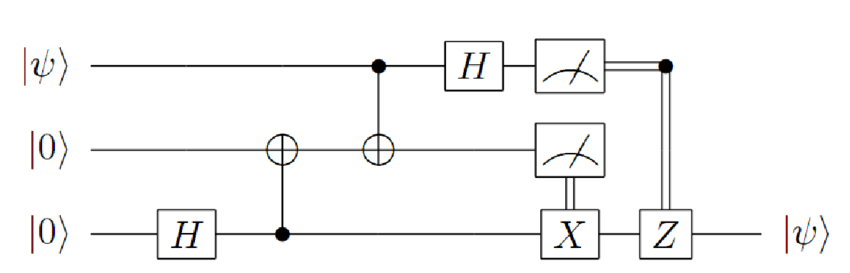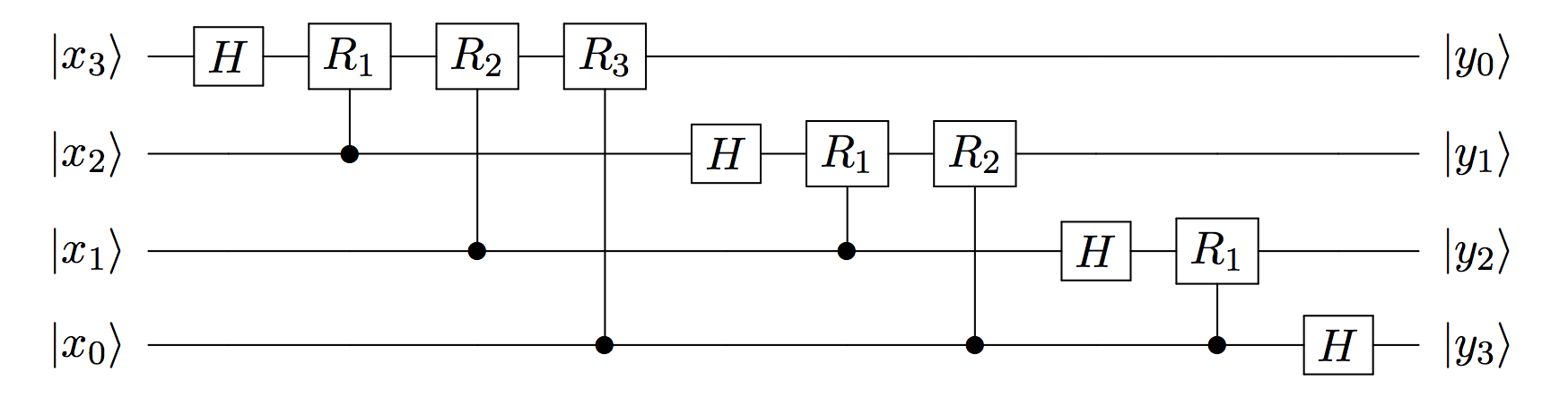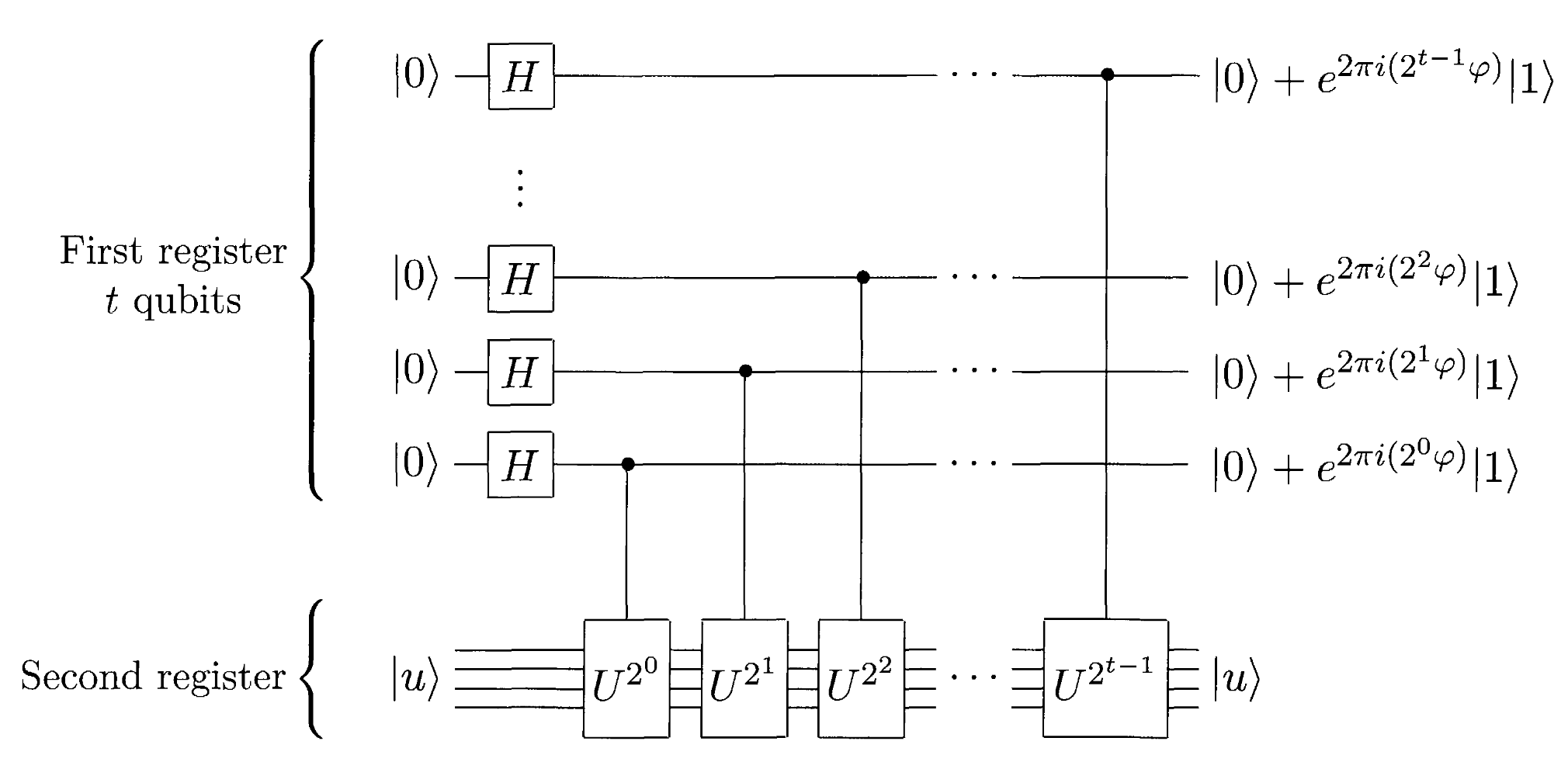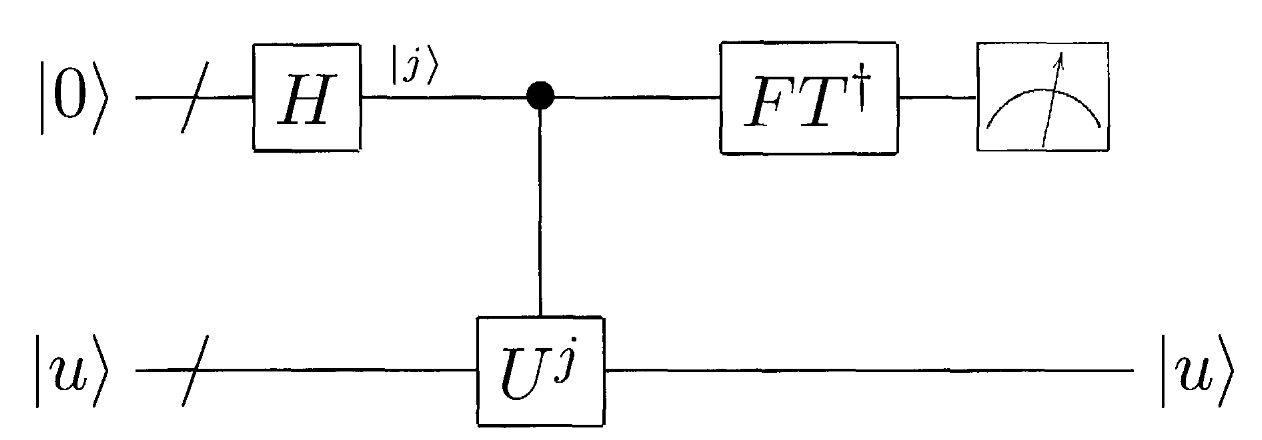## 1. QKD 方案

1. BB84 方案：

Alice 制备一组量子态，制备时随机选取为 X 测量基或 Z 测量基下的本征态 $\vert \pm x\rangle$, $\vert \pm z\rangle$ ，并将这些态发送给 Bob。Bob 则随机选择 X 测量基或 Z 测量基进行测量。之后，双方通过经典信道公开各自的测量基序列，二者测量基一致的部分的结果即可作为共享的密钥。

窃听检测：在测量基相同的部分中，选出一小部分结果公开并比对。如果存在 Eve，由于其无法提前知道 Alice 的制备基，比对结果中会有 1/4 的结果 Alice 与 Bob 不一致，由此可发现窃听。

2. B92 方案：

Alice 制备一组量子态，随机制备为 $\vert +x\rangle$ 作为 0 或者 $\vert +z\rangle$ 作为 1，并发送给 Bob。Bob 依旧随机选取 X 或者 Z 测量基测量。注意到，此时如果在 Z 或 X 测量基下测的本征值为 -1，则 Bob 知道对应的态一定为 0 或者 1，否则记为 $?$。结束后只需要 Bob 告诉 Alice 自己得到的确定态的序号，二者即可将这些态作为共享密钥。

窃听检测：A，B 双方公开部分 Bob 指定的得到确定结果的非 $?$ 测量，如果存在 Eve，则两者会存在一定比例的结果不一致。该错误率为 1/4。这一概率，需要详细列出包含 A 发送态，E 测量基，E 测量结果（E 发送给 B 的态可能需要根据测量结果修改），B 测量基，B 测量结果的五层二叉树，以最后结果中 B 确认的态的数目为分母，结论与 A 态不符的部分为分子得出。其次，在此之前还需注意，Bob 可确定的态（非$?$）的比例应占 1/4 左右，否则可能已存在窃听者篡改。

PhysRevLett.68.3121

3. E91 方案：

将一系列 EPR pair （$\vert 01\rangle+\vert 10\rangle$） 从一个源分别发送给 Alice 和 Bob。A,B 都随机选择测量基测量，我们将其测量方向记为 $\vec{a_i}, \vec{b_i}, i=1,2,3$。其中 Alice 的测量方向分别是 0，45，90 度，而 Bob 的测量方向分别是 45，90，135 度（以垂直粒子运动的平面为基准）。测量完成后，双方公开测量基，测量基相同（a2和b1, a3和b2）的部分，测量结果必定相反，由此可作为密钥。

窃听检测：我们定义 $E(\vec{a_i},\vec{b_j})=P_{++}+P_{--}-P_{+-}-P_{-+}$，该量描述了两个测量方向的相关性。由量子力学可知 $E(\vec{a_i},\vec{b_j})=-\vec{a_i}\cdot\vec{b_j}$。定义 $S=E(a_1,b_1)-E(a_1,b_3)+E(a_3,b_1)+E(a_3,b_3)$，对于完全纠缠态，我们有 $E=-2\sqrt{2}$。 测量基选择不同的部分，可用来计算 S 是否违背贝尔不等式，从而确保 EPR pair 的纠缠依旧充分，保证了无窃听者替换了粒子。也即如果有窃听者测量，干扰纠缠态的话，则 $\vert S\vert\leq \sqrt{2}$，满足Bell 不等式定域性。

PhysRevLett.67.661

## 2. Dense Coding

• 量子密集编码线路：• 量子隐形传态线路：## 4. 量子安全直接通讯方案

QSDC 无需首先通过量子信道协商和确认密钥再在经典信道加密传输信息，而是直接将信息本身在量子信道内传输。

PhysRevA.65.032302

## 5. 量子秘密共享方案

QSS 可使得只有几方同时认同参与才能使用完整的密钥。比如通过协商的方式，Alice 可以保证自己的密钥，只有 Bob 和 Charlie 同时同意时才能使用。也即该密钥可由 Alice 使用，也可以由 Bob+Charlie 使用。

PhysRevA.59.1829

## 6. Shor 算法

Shor 算法是量子部分解决阶问题和经典部分转化为合数分解问题的结合，其总体上可以在多项式时间解决合数分解问题，实现可能的指数加速。

1. 若 N 为偶数，直接返回 2.
2. 判断是否存在 a,b，使得 $a^b=N$，若存在，返回 a。
3. 随机选取 $1\leq x\leq N-1$，若 $\gcd(x,N)>1$，返回该公约数。
4. 使用量子线路，计算 x 关于 N 的阶数 r。
5. 若 r 为偶数，且 $x^{r/2}\neq -1\;\mod N$，进入6，否则重新进行3。
6. 计算 $\gcd(x^{r/2}-1,N)$$\gcd(x^{r/2}+1,N)$ ，检查其中哪个是 N 的因子，并返回该公约数。

• 所有的求公约数 gcd 的操作，都需要用辗转相除法，用来保证多项式时间。
• 第二步，需要依次尝试 $N^{1/k}$，直到 $k=\lceil \log_2N\rceil$，所以也是多项式时间。
• 只有第 4 步需要用到量子线路，其他都是经典算法。事实上，可以证明，合数分解和阶数寻找的复杂度多项式等价。（两问题可互相解决）
• 之所以不需要判断 $x^{r/2}=1\;\mod N$，是因为 r 作为阶数的定义，是最小的使 x 指数余1的整数，因此 $r/2$ 指数不可能余1。
• 算法保证了核心检查步骤 5，至少具有 $3/4$ 的成功概率。

## 7. Grover 算法

1. 原始算法步骤

1. 相位匹配

quant-ph/0107013

1. 龙算法

Grover 算法通过两次镜面使得量子态接近标记态，但是无法保证最优搜索次数时测量得到标记态的概率为 1。Long 算法进行了改进，利用上述的旋转代替镜面反转，通过调节旋转的 phase，可以使得指定搜索次数后标记态出现概率达到 1.

PhysRevA.64.022307

## 8. Steane code

Steane code 由 7 个物理比特编码一个逻辑比特。只考虑单比特错误，则有 $3*7+1=22$ 种情况。其 stablizer 生成元为 $M_1=X_4X_5X_6X_7, M_2=X_2X_3X_6X_7, M_3=X_1X_3X_5X_7$, $N_1=Z_4Z_5Z_6Z_7, N_2=Z_2Z_3Z_6Z_7, N_3=Z_1Z_3Z_5Z_7$. 对应的逻辑态为

## 10. CSS code

CSS code 的逻辑比特组成为

Steane 码是 CSS code 的一个具体实现，其需要的经典码 $C_1$ 的 H 矩阵为

## 12. 相位估计算法其中 $R_n=Diag[1, e^{\pi i/2^n}]$. 注意线路图最后省略了一个交换线路，生成的 y 的顺序正好是相反的。